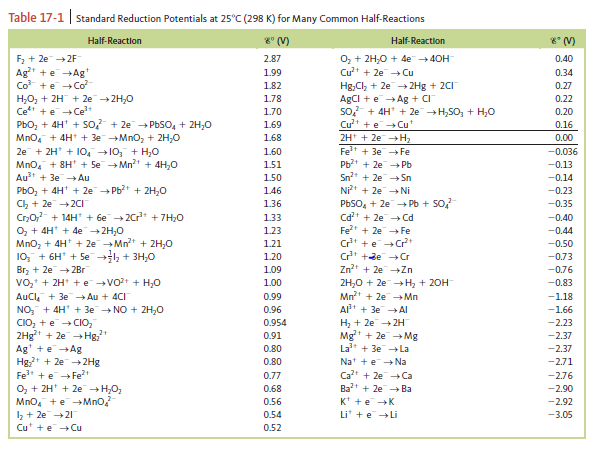# Problem: For the following half-reaction, ε° = —2.07 V:AlF63-(aq) + 3e - → Al(s) + 6F -(aq)Using data from the following table, calculate the equilibrium constant at 25°C for the reactionAl 3+(aq) + 6F -(aq) ⇌ AlF63-(aq)    K = ?

###### FREE Expert Solution

Overall reaction:     Al3+(aq) + 6F -(aq) ⇌ AlF63-(aq)

Step 1: Identify the anode and the cathode in the reaction.

AlF63-(aq) + 3e → Al(s) + 6F -(aq)                      E° = 2.07 V               ↓ E° → oxidation → anode

(since we also need AlF63-(aq)  on the product side)

Al3+(aq) + 3 e- Al(s)                                          E° = – 1.66 V               ↑ E° → reduction → cathode

Step 2: Write the overall reaction and determine the number of electrons transferred.

lose electrons oxidation → anode
gain electrons reduction → cathode

Al(s) + 6 F -(aq)  → AlF63-(aq) + 3e -
Al3+(aq) + 3 e- Al(s)
___________________________
Al3+(aq) + 6 F -(aq)  → AlF63-(aq)

# of electrons transferred = 3 e­-

Step 3: Calculate the cell potential of the reaction.

cell = 0.41 V

Step 4: Calculate the equilibrium constant (K) using the Nernst Equation.

82% (64 ratings)###### Problem Details

For the following half-reaction, ε° = —2.07 V:

AlF63-(aq) + 3e → Al(s) + 6F -(aq)

Using data from the following table, calculate the equilibrium constant at 25°C for the reaction

Al 3+(aq) + 6F -(aq) ⇌ AlF63-(aq)    K = ?Frequently Asked Questions

What scientific concept do you need to know in order to solve this problem?

Our tutors have indicated that to solve this problem you will need to apply the Cell Potential concept. If you need more Cell Potential practice, you can also practice Cell Potential practice problems.

What professor is this problem relevant for?

Based on our data, we think this problem is relevant for Professor Rothberg's class at UR.

What textbook is this problem found in?

Our data indicates that this problem or a close variation was asked in Chemistry: An Atoms First Approach - Zumdahl Atoms 1st 2nd Edition. You can also practice Chemistry: An Atoms First Approach - Zumdahl Atoms 1st 2nd Edition practice problems.